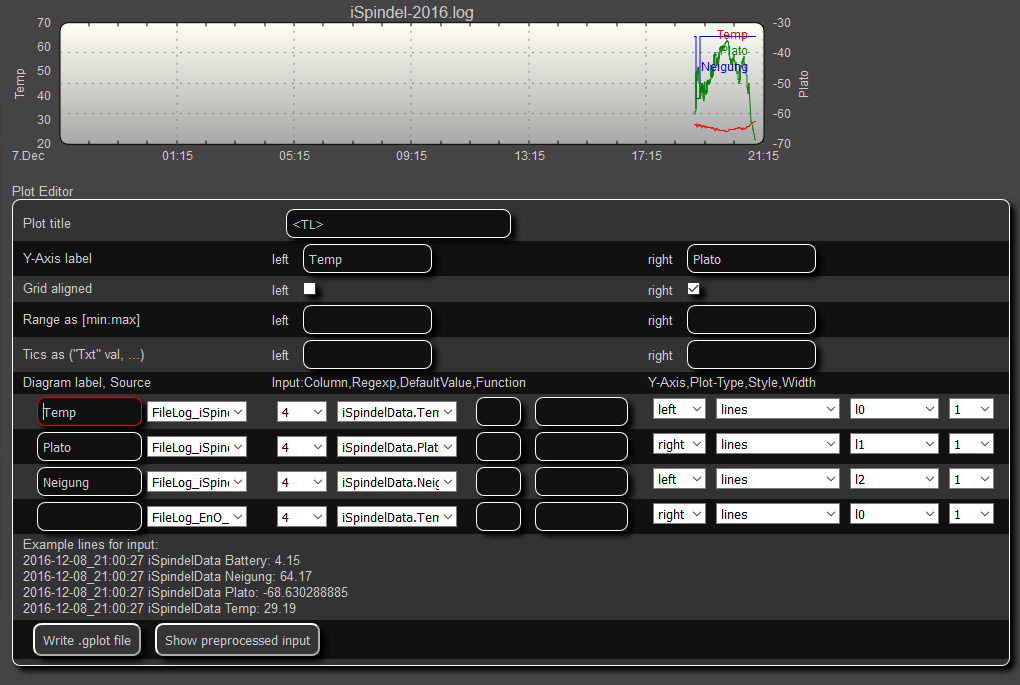DIY electronic Hydrometer

ISpindel can transfer the data to a local FHEM server from where it can be visualized and further processed (for example, to control the fermentation temperature). FHEM expects data by Http Get request:

http://server:8083/fhem?cmd.Test=set%20iSpindel%2011.5%2022.3%203.3&XHR=1

The target host and port (server: 8083) as well as the name of the spindle (here “iSpindel”) must be set in the configurationUI of the spindle. All data to be transferred are transferred in the Url with a Get. The order is tilt - temperature battery. Spaces must be replaced with% 20.

In FHEM (ie fhem.cfg), a device with the name of the spindle (here: “iSpindel”) and a bit of Perl logic must be created in order to split the data record into the individual values. The resulting values ​​are assigned to the device iSpindelData and can be further processed from there.

define iSpindel dummy
define iSpindelData dummy

define iSpindel_Notify notify iSpindel {  \
my \$Neigung=\$x;; \
my \$Temp=\$x;; \
my \$Battery=\$x;; \
my \$Plato=CalcPlato(\$Neigung, \$Temp);; \
}

define iSpindel_Values readingsGroup iSpindelData:Temp iSpindelData:Neigung iSpindelData:Battery iSpindelData:Plato
attr iSpindel_Values room Bier

define FileLog_iSpindel FileLog ./log/iSpindel-%Y.log iSpindelData.*
attr FileLog_iSpindel logtype text

The function CalcPlato (slope, temperature) can be stored in 99_MyUtils.pm:

sub CorrectPlato(\$\$)  #(P, T)
{
my \$mPlato=shift;
my \$Temp=shift;
my @k=(56.084,   -0.17885,   -0.13063);
if (\$mPlato>=5) { @k=(69.685,    -1.367,      -0.10621);};
if (\$mPlato>=10){  @k=(77.782,    -1.7288,    -0.10822);};
if (\$mPlato>=15){  @k=(87.895,    -2.3601,    -0.10285);};
if (\$mPlato>=20){  @k=(97.052,    -2.7729,    -0.10596);};

my \$cPlato=\$k+\$k*\$Temp+\$k*\$Temp*\$Temp;
return \$mPlato-\$cPlato/100;
}

sub CalcPlato(\$\$)   #(Angle, T)
{
my \$tilt=shift;
my \$Temp=shift;
# Get this from Excel Calibration at 20 Degrees
my \$mPlato=0.00438551*(\$tilt)*(\$tilt) + 0.13647658*(\$tilt) - 6.968821422;
return CorrectPlato(\$mPlato,\$Temp);
}

The formula for \$mPlato must be taken from the calibration sample sheet. CorrectPlato () is also used to perform a temperature correction of the Platower (requires calibration at 20 degrees). The temperature correction is valid for the range of 5 to 30 degrees Celsius.

Lastly, only the diagram for the spindle values ​​has to be defined; This is very easy with the FHEM -GPlot editor and could look something like this:Definition iSpindel Diagram

attr WEB endPlotNow 1